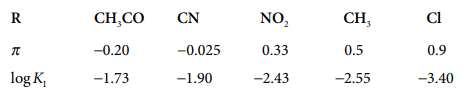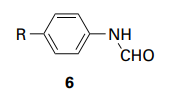×
Get Full Access to Atkins' Physical Chemistry - 11 Edition - Chapter 14e - Problem P14e.1
Get Full Access to Atkins' Physical Chemistry - 11 Edition - Chapter 14e - Problem P14e.1

×ISBN: 9780198769866 2042

## Solution for problem P14E.1 Chapter 14E

Atkins' Physical Chemistry | 11th Edition

• Textbook Solutions
• 2901 Step-by-step solutions solved by professors and subject experts
• Get 24/7 help from StudySoup virtual teaching assistantsAtkins' Physical Chemistry | 11th Edition

4 5 1 276 Reviews
13
5
Problem P14E.1

The binding of nonpolar groups of amino acid to hydrophobic sites in the interior of proteins is governed largely by hydrophobic interactions.

(a) Consider a family of hydrocarbons R-H. The hydrophobicity constants, $$\pi$$, for $$\mathrm{R}=\mathrm{CH}_{3}$$, $$\mathrm{CH}_{2} \mathrm{CH}_{3}$$,$$\left(\mathrm{CH}_{2}\right)_{2} \mathrm{CH}_{3}$$,$$\left(\mathrm{CH}_{2}\right)_{3} \mathrm{CH}_{3}$$, and $$\left(\mathrm{CH}_{2}\right)_{4} \mathrm{CH}_{3}$$ are, respectively, 0.5,1.0,1.5,2.0, and 2.5. Use these data to predict the $$\pi$$ value for $$\left(\mathrm{CH}_{2}\right)_{6} \mathrm{CH}_{3}$$.

(b) The equilibrium constants $$K_{I}$$ for the dissociation of inhibitors (6) from the enzyme chymotrypsin were measured for different substituents R:Plot $$\log K_{I}$$ against $$\pi$$. Does the plot suggest a linear relationship? If so, what are the slope and intercept to the $$\log K_{I}$$ axis of the line that best fits the data?

(c) Predict the value of $$K_{\mathrm{I}}$$ for the case R=H.Text Transcription:

pi

R=CH_3

CH_2 CH_3

(CH_2)_2 CH_3

(CH_2)_3 CH_3

(CH_2)_4 CH_3

(CH_2)_6 CH_3

K_I

log K_I

Step-by-Step Solution:
Step 1 of 3

o*2 c lo-* = (O,lO)(35,nr_) - o 'Ornl t35.s x] *d 5l Z" * ""4 il*s -- ,^2v ,r wt* Lrt h t &r *- * L 4 nro "*z{0"t--n*{-il"* = {oq}* (

Step 2 of 3

Step 3 of 3

##### ISBN: 9780198769866

The full step-by-step solution to problem: P14E.1 from chapter: 14E was answered by Aimee Notetaker, our top Chemistry solution expert on 04/25/22, 03:45PM. Since the solution to P14E.1 from 14E chapter was answered, more than 201 students have viewed the full step-by-step answer. The answer to “?The binding of nonpolar groups of amino acid to hydrophobic sites in the interior of proteins is governed largely by hydrophobic interactions.(a) Consider a family of hydrocarbons R-H. The hydrophobicity constants, $$\pi$$, for $$\mathrm{R}=\mathrm{CH}_{3}$$, $$\mathrm{CH}_{2} \mathrm{CH}_{3}$$,$$\left(\mathrm{CH}_{2}\right)_{2} \mathrm{CH}_{3}$$,$$\left(\mathrm{CH}_{2}\right)_{3} \mathrm{CH}_{3}$$, and $$\left(\mathrm{CH}_{2}\right)_{4} \mathrm{CH}_{3}$$ are, respectively, 0.5,1.0,1.5,2.0, and 2.5. Use these data to predict the $$\pi$$ value for $$\left(\mathrm{CH}_{2}\right)_{6} \mathrm{CH}_{3}$$. (b) The equilibrium constants $$K_{I}$$ for the dissociation of inhibitors (6) from the enzyme chymotrypsin were measured for different substituents R: Plot $$\log K_{I}$$ against $$\pi$$. Does the plot suggest a linear relationship? If so, what are the slope and intercept to the $$\log K_{I}$$ axis of the line that best fits the data?(c) Predict the value of $$K_{\mathrm{I}}$$ for the case R=H. Text Transcription:piR=CH_3CH_2 CH_3(CH_2)_2 CH_3(CH_2)_3 CH_3(CH_2)_4 CH_3(CH_2)_6 CH_3K_Ilog K_I” is broken down into a number of easy to follow steps, and 128 words. This textbook survival guide was created for the textbook: Atkins' Physical Chemistry, edition: 11. This full solution covers the following key subjects: . This expansive textbook survival guide covers 327 chapters, and 1120 solutions. Atkins' Physical Chemistry was written by Aimee Notetaker and is associated to the ISBN: 9780198769866.

## Discover and learn what students are asking

Calculus: Early Transcendental Functions : Multiple Integration
?In Exercises 1 and 2, evaluate the integral. $$\int_{0}^{2 x} x y^{3} d y$$

Calculus: Early Transcendental Functions : Integration by Tables and Other Integration Techniques
?In Exercises 47-52, verify the integration formula. \(\int \frac{u^{n}}{\sqrt{a+b u}} d u=\frac{2}{(2 n+1) b}\left(u^{n} \sqrt{a+b u}-n a \in

Statistics: Informed Decisions Using Data : Inference about the Difference between Two Medians: Dependent Samples
?In Problems 3–10, use the Wilcoxon matched-pairs signedranks test to test the given hypotheses at the a = 0.05 level of significance. The dependent sa

Chemistry: The Central Science : The Chemistry of Life: Organic and Biological Chemistry
?Identify the carbon atom(s) in the structure shown that has (have) each of the following hybridizations: (a) s p^{3}, (b) sp,

Unlock Textbook Solution